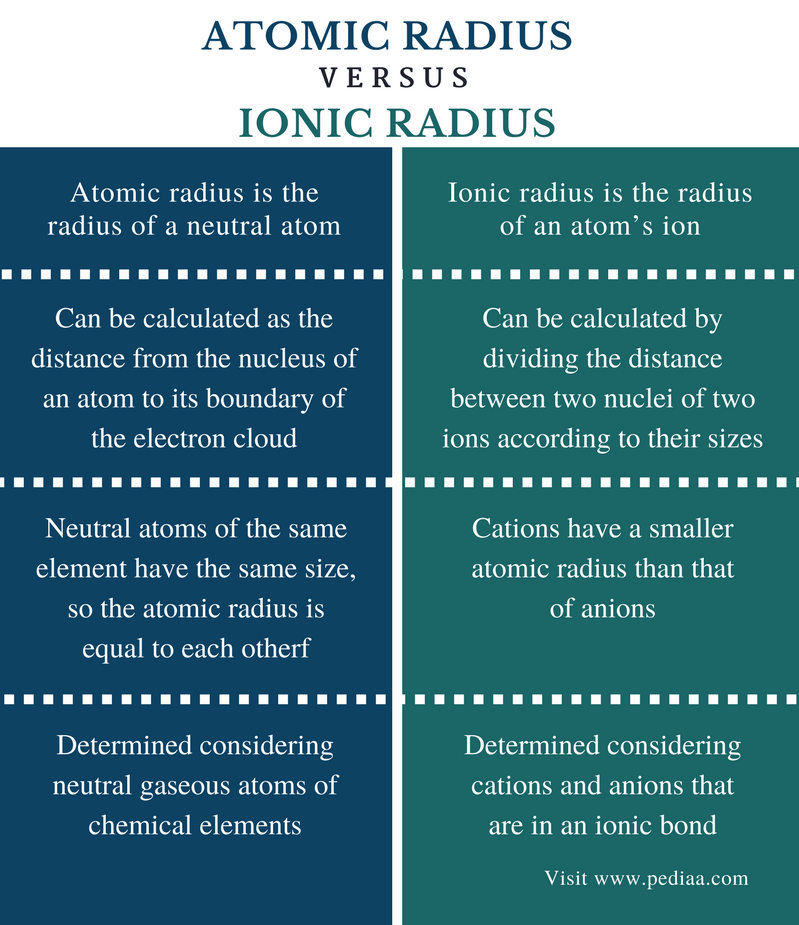### Key Areas Covered

– Definition, Trends in the Periodic Table

– Definition, Trends in the Periodic Table

– Comparison of Key Differences

Key Terms: Atomic Radius, Atoms, Electron Shell, Ionic Radius, IonsAtomic radius is the distance from the nucleus of an atom to its boundary of the electron cloud. In other words, it is the distance from the nucleus to the farthest electron that belongs to that atom. Atomic radius can be defined only for isolated and neutral atoms.

When considering the periodic table of elements, there is a pattern of the atomic radius of elements. Along a period of the periodic table, the atomic number is gradually decreased. The elements in the same period have the same number of electron shells. If the number of electrons present is higher, the attraction between the electrons and the nucleus is also high. In the beginning of the period, there is a less number of electrons present in the outermost orbital. Then the attraction from the nucleus is less. Therefore, the atom is large, and the atomic radius is also large. But when moving along a period, the number of protons in the nucleus is increased along with the number of electrons present in the atom. Therefore, the attraction force between electrons and the nucleus is high. It causes the size of the atom to shrink; then the atomic radius is reduced. Likewise, when moving along a period, the size of the atom is decreased gradually, so is the atomic radius.Figure 1: Comparison of Atomic Sizes

When moving down a group of the periodic table of elements, the atomic radius is increased. After each period, one more electron shell is added to the atom. Therefore, when moving down the group, the size of the atom is increased. The atomic radius is also increased.

But in the d block elements, there is no higher difference between the atomic radii of atoms of two adjacent elements in the same period. This is because the electrons here are added to the same d orbital that is located as an inner orbital. Since the outermost shell remains constant, the atomic radii of those elements do not have considerable differences.

Ionic radius is the radius of an atom’s ion. Ions cannot exist alone. If it is a positively charged ion, it will react with a negatively charged ion (or the opposite) and become a stable neutral compound. This compound is called an ionic compound because it is made out of ionic components. An ionic compound is composed of cations and anions. The cation is smaller in size because a cation is formed by removing one or more electrons from an atom. The anion is large because it has extra electrons that are repelled by the nucleus, resulting in the increase of the distance between the nucleus and the farthest electron of the electron cloud.

The most accurate way to find the ionic radius is to divide the distance between two nuclei of two ions according to their sizes. For example, if an ionic compound is composed of a cation and an anion that has an atomic size which is three times larger, the distance between the two nuclei should be divided by 4 in order to obtain the cation radius.Figure 2: Atomic and Ionic Radii of Some Elements

The ions of the same chemical element can be found in different sizes according to their electrical charges. The most common method to find the ionic radius is the X-ray crystallography. Same as in atomic radius, the ionic radius also has trends in the periodic table. As we move down a group in the periodic table, the ionic radius is increased. This is because a new electron shell is added per each period when we go downward a group. Along a period, the ionic radius is decreased due to the effective positive attraction from the nucleus is increased gradually.

### Calculation

Atomic Radius: Atomic radius can be calculated as the distance from the nucleus of an atom to its boundary of the electron cloud.

Ionic Radius: Ionic radius can be calculated by dividing the distance between two nuclei of two ions according to their sizes.

### Sizes

Atomic Radius: Neutral atoms of the same element have the same size, so the atomic radius is equal to each other.

Ionic Radius: Cations have a smaller atomic radius than that of anions.

### Determination

Atomic Radius: Atomic radius is determined considering neutral gaseous atoms of chemical elements.

Ionic Radius: Ionic radius is determined considering cations and anions that are in an ionic bond (in ionic compounds).

### Conclusion

Atomic radius and ionic radius of chemical elements have trends in the periodic table of elements. The increasing or decreasing of the atomic or ionic sizes along a period or down a group of the periodic table can be explained using the electron configurations of elements. However, there are considerable differences between atomic radius and ionic radius. The main difference between atomic radius and ionic radius is that the atomic radius is the radius of a neutral atom whereas the ionic radius is the radius of an electrically charged atom.

##### References:

1. Helmenstine, Anne Marie. “Here’s What Trends Ionic Radius Follow in the Periodic Table.” ThoughtCo, Available here. Accessed 21 Sept. 2017.
2. Libretexts. “Atomic Radii.” Chemistry LibreTexts, Libretexts, 7 Sept. 2017, Available here. Accessed 21 Sept. 2017.

##### Image Courtesy:

1. “Atomic & ionic radii” By Popnose – Own work (ionic radii from R. D. Shannon (1976). “Revised effective ionic radii and systematic studies of interatomic distances in halides and chalcogenides”. Acta Cryst A32: 751–767. DOI:10.1107/S0567739476001551.) (CC BY-SA 3.0) via Commons Wikimedia
2.”Comparative atomic sizes” By CK-12 Foundation (CC BY-SA 3.0) via Commons Wikimedia